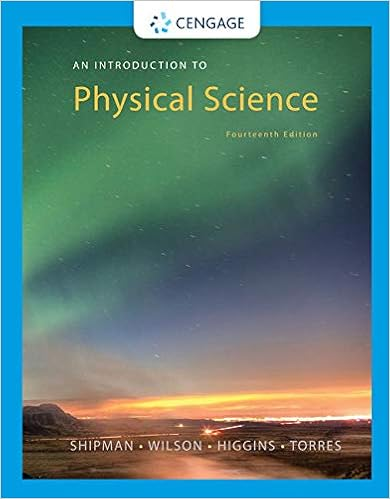# The chlor alkali process produces chlorine cl 2 g in

• Test Prep
• 11
• 95% (22) 21 out of 22 people found this document helpful

This preview shows page 8 - 10 out of 11 pages.

##### We have textbook solutions for you!
The document you are viewing contains questions related to this textbook.The document you are viewing contains questions related to this textbook.
Chapter 13 / Exercise 7
An Introduction to Physical Science
Shipman/WilsonExpert Verified
38.The chlor-alkali process produces chlorine, Cl2(g), in large quantities. What other industrially important substances are produced in this process?A)Na(s), H2(g)D)Na(s), O2(g)B)H2(g), O2(g)E)NaOH(aq), H2(g)C)Hg(l), NaCl(s)Ans: E
Difficulty: M39.Describe, and give reasons for, the fundamental bonding differences between transition metal sulfides and transition metal oxides.
Difficulty: M40.Give two important reasons why there is so much more Na+in the oceans than K+, despite their similar abundances in the earth's crust.
Difficulty: M41.The mass of the atmosphere has been estimated to be 5.2 1021g, while the average molar mass of atmospheric gases is 28.9 g/mol. If the concentration of carbon dioxide inthe atmosphere is 360 ppm by volume, calculate the mass of this gas in the atmosphere, in billions of metric tons, bmt. (1 bmt = 10
.
42.Briefly describe the three main pathways for nitrogen fixation.Ans:
Difficulty: MPage 130
##### We have textbook solutions for you!
The document you are viewing contains questions related to this textbook.The document you are viewing contains questions related to this textbook.
Chapter 13 / Exercise 7
An Introduction to Physical Science
Shipman/WilsonExpert Verified
Chapter 22: The Elements in Nature and Industry43.Lightning, fires, and other atmospheric processes result in the formation of nitric acid, HNO3, from atmospheric N2, O2,and H2O. Write balanced chemical equations to represent the three reactions involved in the formation of HNO3by this route.
g)
g)
g)
44.The leaching of gold can be represented by the equation4Au(s) + O2(s) + 8CN(aq) + 2H2O(l) 4Au(CN)2(aq) + 4OHIn this reaction, identify the oxidant, the reductant and the oxidation numbers of the elements which change.(aq)
•••